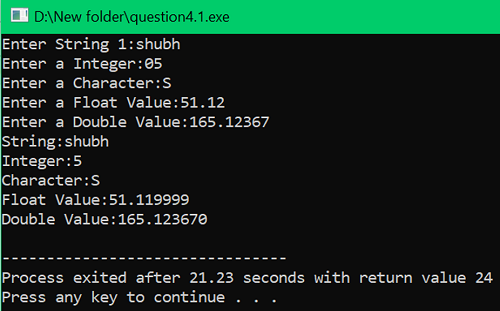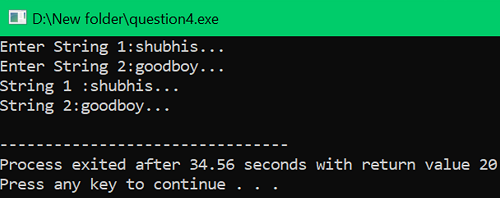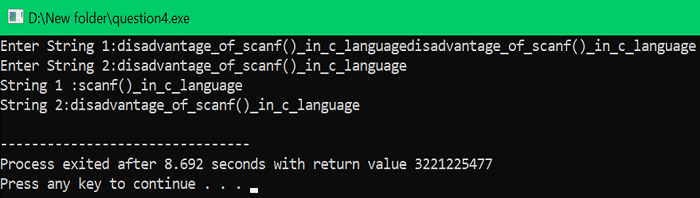# Disadvantages of scanf() function in C language

In this tutorial, we will learn about the scanf() function and its major disadvantages.
Submitted by Shubh Pachori, on July 03, 2022

scanf() function is used to read any input that is given by the user and store it in the specified variable. scanf() function only reads and store the input if it is the same type of the given format specifier.

Consider the below example which gives us a great overview of the scanf() function's working.

Example:

```#include <stdio.h>

int main()
{
char str; //string
int i; //integer variable
char c; // character variable
float f; // float variable
double d; // double variable

printf("Enter String 1:");
// command to clear the keyboard memory
fflush(stdin);
scanf("%s", str);

printf("Enter a Integer:");
fflush(stdin);
scanf("%d", &i);

printf("Enter a Character:");
fflush(stdin);
scanf("%c", &c);

printf("Enter a Float Value:");
fflush(stdin);
// scanf() reading a float value
scanf("%f", &f);

printf("Enter a Double Value:");
fflush(stdin);
// scanf() reading a double value
scanf("%lf", &d);

printf("String:%s\n", str);
printf("Integer:%d\n", i);
printf("Character:%c\n", c);
printf("Float Value:%f\n", f);
printf("Double Value:%lf\n", d);

return 0;
}
```

Output:In the scanf() function there is a problem with buffer overflow. Buffer overflow is a problem it occurs when the size of the information written in a memory location exceeds the memory limit that is allotted to it. In simple words, it is a problem of excessive input than the allocated memory of a declared variable. In this, there is a messing up of input that is stored in the variable using the scanf() function and it prints the useless values and incorrect output.

Consider the below example, there are two strings declared of 10 characters each. So, there are two memory locations of 10 bytes each allocated to them. In the first output (Output 1) we have given an input of exactly 10 characters so we can see that there is no incorrect or messed-up output. But if we input more characters than the allocated memory then it will mess up the input and produce wrong and incorrect output (Output 2).

Example to demonstrate the buffer overflow problem in scanf() function

```#include <stdio.h>

int main()
{
char str1; // length of 10 characters
char str2; // length of 10 characters

printf("Enter String 1:");
scanf("%s", str1);

printf("Enter String 2:");
scanf("%s", str2);

printf("String 1 :%s\n", str1);
printf("String 2:%s\n", str2);

return 0;
}
```

Output 1:In the above output, we can see there is no difference in the input string and output string.

Output 2:In the above output, there is a difference between the input string and the output string.